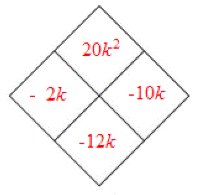### Home > INT2 > Chapter 4 > Lesson 4.1.3 > Problem4-28

4-28.

Factor the following expressions, if possible.

1. $k^2-12k+20$

Use an area model and a Diamond Problem.

 $-2$ $-2k$ $20$ $k$ $k^2$ $-10k$ $k$ $-10$1. $6x^2+17x−14$

$(2x+7)(3x−2)$

1. $x^2-8x+16$

1. $9m^2-1$

Insert a $0m$ between the $9m^2$ and the $−1$.

1. The expressions in parts (a) through (c) are trinomials while part (d) is a binomial, yet they are all quadratic expressions. What makes each of them a quadratic? Justify your responses.

What is the highest power of the variable in each of the problems?